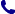• Kontakt
• Newsletter Zapisz się po informacje o nowościach i specjalnych ofertach. Administrator danych: ABE-IPS sp. z o.o.
Wybierz interesujące Cię kategorie
Wyrażam zgodę na przetwarzanie moich danych osobowych w celach marketingowych, tym samym akceptuję Regulamin Newslettera i Politykę prywatności.
Wyrażam zgodę na przesyłanie informacji handlowych drogą elektroniczną.
Wypisz się
• Klienci instytucjonalni
• Dostawa
• O nas
Dostęp on-line

# Książki

0.00 PLN
Schowek (0)
 Schowek jest pusty

## Methods of Mathematical Modelling

 Autorzy Wydawnictwo Springer Nature Customer Service Center GmbH Data wydania 01/09/2015 Wydanie Pierwsze Liczba stron 305 Forma publikacji książka w miękkiej oprawie Poziom zaawansowania Dla profesjonalistów, specjalistów i badaczy naukowych Język angielski ISBN 9783319230412 Kategorie Rachunek różniczkowy i formuły, Rachunek wariacyjny, modelowanie matematyczne
Zapytaj o ten produkt
 E-mail Pytanie
Do schowka

## Opis książki

This book presents mathematical modelling and the integrated process of formulating sets of equations to describe real-world problems. It describes methods for obtaining solutions of challenging differential equations stemming from problems in areas such as chemical reactions, population dynamics, mechanical systems, and fluid mechanics. Chapters 1 to 4 cover essential topics in ordinary differential equations, transport equations and the calculus of variations that are important for formulating models. Chapters 5 to 11 then develop more advanced techniques including similarity solutions, matched asymptotic expansions, multiple scale analysis, long-wave models, and fast/slow dynamical systems. Methods of Mathematical Modelling will be useful for advanced undergraduate or beginning graduate students in applied mathematics, engineering and other applied sciences. "The text is well written. The authors have provided a clear and concise presentation of many important topics in a way that should be accessible to students following a first course in differential equations. ... More advanced students could easily learn a significant amount of useful mathematics reading the text independently. ... Methods of Mathematical Modelling is a welcome addition to the SUMS series and should prove to be useful for many instructors and students." (Jason M. Graham, MAA Reviews, maa.org, February, 2016) "The purpose of this text is to introduce the reader to the art of mathematical modeling ... . The book provides an account of a number of useful for mathematical modelling techniques which are illustrated with examples and complemented with problems for self study." (Yuriy V. Rogovchenko, zbMATH 1333.00025, 2016)

Methods of Mathematical Modelling

## Spis treści

Rate equations.- Transport equations.- Variational principles.- Dimensional scaling analysis.- Self-similar scaling solutions of differential equations.- Perturbation methods.- Boundary layer theory.- Long-wave asymptotics for PDE problems.- Weakly-nonlinear oscillators.- Fast/slow dynamical systems.- Reduced models for PDE problems.- Modelling in applied fluid dynamics.

## Polecamy również książki801 777 223